# Calculate Birthdays Anniversary / Jubilee from birthday date.

643
15
12-16-2022 03:37 AM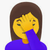by
New Contributor III

Hello every one,

my question is quite straightforward: I want to enter a birthday date and have the Birthday jubilee, f.i. the 18th, the 20th, 30th birthdays and so on , automatically calculated.

I used the following formula (from here Decimal date time—ArcGIS Survey123 | Documentation😞

• 18th Anniversary: date(decimal-date-time(\${Geb_Datum}) + (365.25*18))
• 20th Anniversary: date(decimal-date-time(\${Geb_Datum}) + (365.25*20))

But when I enter a Birthday, the calculation seems to have a 1-Day error, but only in some Jubilees.

Birthday date 01.12.2020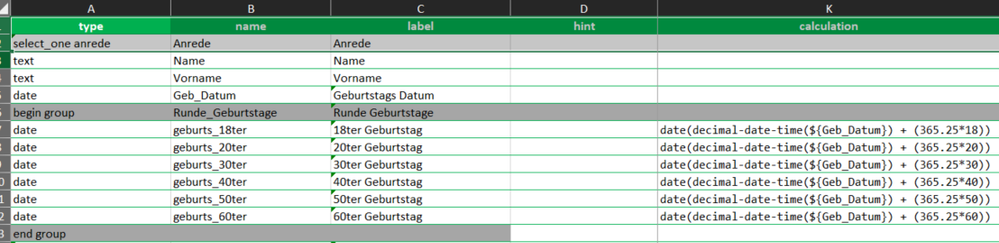Birthday date 01.12.2020Very strange is that depending on the date entered, the same calculation seems to be right:

Birthday date 01.11.1979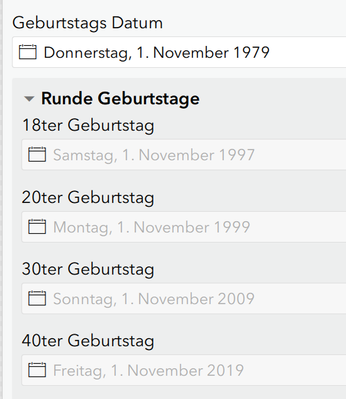Tags (1)
15 RepliesOccasional Contributor III

Great question! I'm wondering if it's a leap-year issue.

I found this online; let me know if this fixes your calculations.

Leap years — years with an extra day at the end of February — were invented as a way to keep calendar years (which are normally 365 days long) and astronomical years (which are 365.2425 days long) in sync with one another.by
New Contributor III

Hello Michelle,

thank You for your suggestion, I tried it and guess what? the results were inverted. The Birthday date 01.12.2020 previosuly wrong, was now right. And the birthday date 01.11.1979 previously right is now wrong.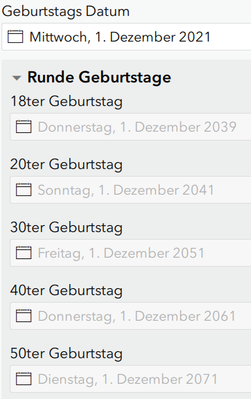Any other ideas?

Thank You very much!!by
New Contributor III

Hello guys,

@JamesTedrick You are en expert in Survey123 Solutions, have you ever came acrosso such a case?

I very much appreciate your help.

Thanks in advance and my kindest regards,

Dorella

THANKS!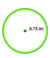Occasional Contributor III

It was a problem with the leap year.

(I am not 100% sure on how exactly this works, but here's my thought process to solve this)

Specifically, if the year input is a leap year, you have to account for the difference by subtracting one day.

`if(format-date(\${Ex8},'%Y') mod 4 = 0, date(decimal-date-time(\${Ex8}) + ((365.25*18)- 1)), date(decimal-date-time(\${Ex8}) + ((365.25*18))))Formula explanation: if the input year is evenly divisible by 4, subtract one day for a leap year, otherwise add as normal.`

This yields the following for December 1, 2020, and for November 1, 1979, as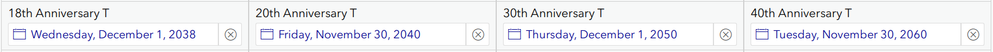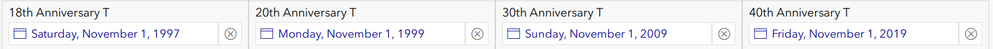You'll notice that for leap years, the 20th and 40th anniversaries are showing up incorrectly. That is (as best I can tell), because those years are evenly divisible by four also.

To fix this, just use your original formula for those fields.

`date(decimal-date-time(\${Geb_Datum}) + (365.25*20))`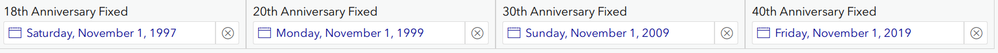Type Name Label Calculation date Ex8 Example 8 date Ann18Fix 18th Anniversary Fixed if(format-date(\${Ex8},'%Y') mod 4 = 0, date(decimal-date-time(\${Ex8}) + ((365.25*18)- 1)), date(decimal-date-time(\${Ex8}) + ((365.25*18)))) date Ann20Fix 20th Anniversary Fixed date(decimal-date-time(\${Ex8}) + (365.25*20)) date Ann30Fix 30th Anniversary Fixed if(format-date(\${Ex8},'%Y') mod 4 = 0, date(decimal-date-time(\${Ex8}) + ((365.25*30)- 1)), date(decimal-date-time(\${Ex8}) + ((365.25*30)))) date Ann40Fix 40th Anniversary Fixed date(decimal-date-time(\${Ex8}) + (365.25*40))

Hope this helps!by
New Contributor III

Dear Alfred,

I tried your suggested solution but unfortunately it didn't work, or at least something in the logic needs to be adjusted.
When I enter my first two TEST birthday dates (01/01/2020 and 11/01/1979) your solution adapts perfectly, but when I randomly choose another birthday date it sometimes doesn't work.I hereby also attach the xls form screenshot:I think there must be a way to correct this, perhaps by converting the result to a string and then applying logic that if the number representing the DAY is different than the number in the "Birthday" field, should then be replaced back into a date field, but that's way too complex for me. And anyway, I still can't believe Esri hasn't come across such a problem yet. Maybe not for anniversaries, but for example to calculate when an inspection needs to be repeated in the future or when an object needs to be changed or checked in the future... I'm not sure I can explain myself clearly enough, I apologize for that.

Thank you for your help and I look forward to your opinion.

Best regards!Occasional Contributor III

Hi DoZ,

That is really strange.

I took another look, and it looks like it's specifically having issues with the year AFTER a leap year, as well.

Luckily, that's an easy fix.

`if(format-date(\${Ex8},'%Y') mod 4 = 0 or format-date(\${Ex8},'%Y') mod 4 = 1 , date(decimal-date-time(\${Ex8}) + ((365.25*18)- 1)), date(decimal-date-time(\${Ex8}) + ((365.25*18))))`

This formula checks to see if it's a leap year or the year after by seeing if it is evenly divisible by 4, or if it has a remainder of 1. (I checked each year from 1977 to 2010, so it should be good)

1977-1981 for proof: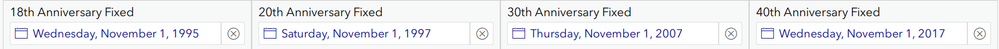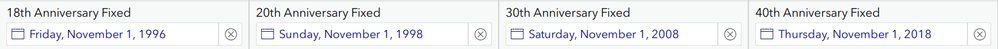Type Name Label Calculation date Ex8 Example 8 date Ann18Fix 18th Anniversary Fixed if(format-date(\${Ex8},'%Y') mod 4 = 0 or format-date(\${Ex8},'%Y') mod 4 = 1 , date(decimal-date-time(\${Ex8}) + ((365.25*18)- 1)), date(decimal-date-time(\${Ex8}) + ((365.25*18)))) date Ann20Fix 20th Anniversary Fixed date(decimal-date-time(\${Ex8}) + (365.25*20)) date Ann30Fix 30th Anniversary Fixed if(format-date(\${Ex8},'%Y') mod 4 = 0 or format-date(\${Ex8},'%Y') mod 4 = 1 , date(decimal-date-time(\${Ex8}) + ((365.25*30)- 1)), date(decimal-date-time(\${Ex8}) + ((365.25*30)))) date Ann40Fix 40th Anniversary Fixed date(decimal-date-time(\${Ex8}) + (365.25*40))

Like you say, it's strange that leap years aren't accounted for.

Hopefully this helps!by
New Contributor III

Dear Alfred,

I thank You so much for your suggestion. I will be trying this during this week and let you know how it worked. I am also sure it has to do with the leap year, only I find it SO STRANGE that ArcGIS didn´t even consider/mention such a problem. I mean if you read the description on the Blog of Mr. Chivite about Dates and Time, leap years are not even mentioned....

Thanks you again, I will come back to You as soon as i tested it out.

Kindest regards

😊by
New Contributor III

Hi Alfred,

I tried it out but please take a look at the Birthday date 1st of November for the 18th Anniversary with the formula:

if(format-date(\${Geb_Datum},'%Y') mod 4 = 0 or format-date(\${Geb_Datum},'%Y') mod 4 = 1, date(decimal-date-time(\${Geb_Datum}) + ((365.25*18)- 1)), date(decimal-date-time(\${Geb_Datum}) + ((365.25*18))))

This formula worked good for years: 1987, 1988,1989, 1990, 1991 till 2022 showing the 1st of November,

but for the years 1986, 1985, 1984, 1983, 1982, 1981 is showing 31st of October

It seems unfortunally there are no leap years involved in this logic...

Leap years were: 1980 1984 1988 1992 1996

Then it get´s more interesting because for year 1980 going back to 1958 it is showing again the correct 1st of November BUT from year 1957 backwards is showing again the wrong date of 31st of October and also the 50th anniversary show a different date..

I am getting so upset! I can´t seem to find a logic...   😥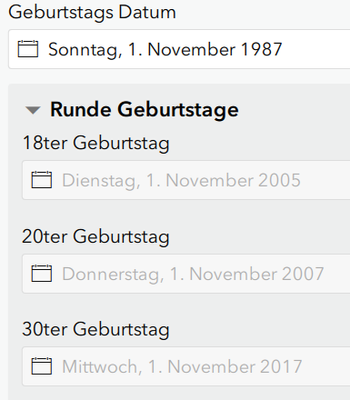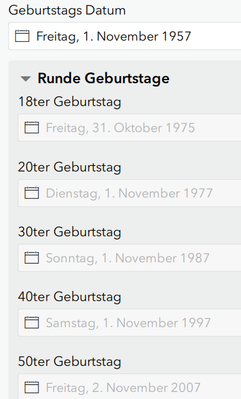by
New Contributor III

Hi Alfred,

it´s me again! So I tried something different....

I converted the birthday date into an integer and added 18 to get the year in which the person will celebrating the 18th birthday.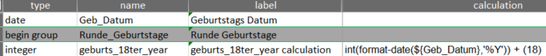After this I created a TEXT field where I take the Day and Month from the birthday´s field and add to it the calculated year as a string. Now I have the correct day and Month and the year in which the person will be 18 years old.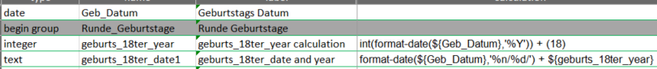After this I just need to convert everything back into a date field: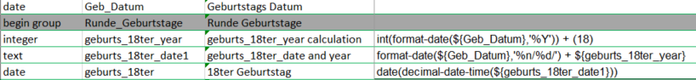I am aware of the fact that I will need 2 extra fields for each anniversary, but I intend to set those fields as hidden and with bind::esri:fieldType as null these will not be published to the feature Service.

What do you think? 😊 maybe is not neat...but at least i avoid the leap year problem.

Regards